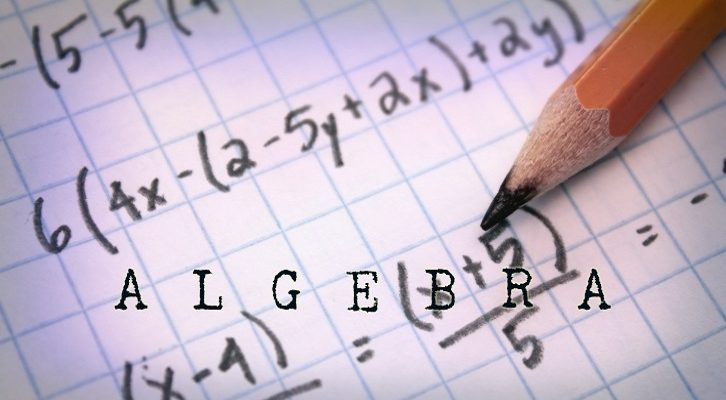# Inequalities – Two more questions

1. (|x| – 2) ( x + 5) < 0. What is the range of values x can take?2. a and b are roots of the equation x^2 – px + 12 = 0. If the difference between the roots is at least 12, what is the range of values p can take?

Batches starting in Chennai for CAT 2013. Weekend batches @ Anna Nagar from 27th October, @ Mylapore from 10th November, @ Velachery from 28th October

Solutions to the above questions

1. (|x| – 2) ( x + 5) < 0 –

This can be true in two scenarios

Scenario I – (|x| – 2)  < 0 and ( x + 5) > 0
Or |x| < 2 and x > -5.This gives us the range (-2,2)

Scenario II – (|x| – 2)  > 0 and ( x + 5) < 0
Or |x| > 2 and x < -5. This gives us the range (-Infinity, -2)

So, the overall range is (-infinity, -2) or (-2,2)

2. a and b are roots of the equation x^2 – px + 12 = 0. If the difference between the roots is at least 12, what is the range of values p can take?

The roots are a and b
a + b = p ab = 12
(a + b )^2 = p^2
(a -b)^2 = (a + b ) ^2 – 4ab
=> (a-b) ^2 = p^2 – 12*4 = p^2 – 48

If |a-b| > 12 { Difference between the roots is at least 12}
then, (a-b)^2 > 144

p^2 – 48 > 144
p^2 > 192
P > 8sqrt(3) or P < -8 sqrt(3)

### Related posts

1.rajput says:
2.Bee says: